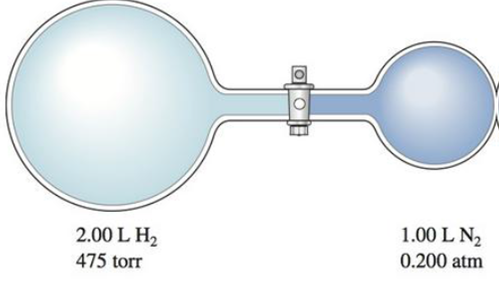# Consider the flasks in the following diagram. What are the final partial pressures of H 2 and N 2 after the stopcock between the two flasks is opened? (Assume the final volume is 3.00 L.) What is the total pressure (in torr)?### Chemistry: An Atoms First Approach

2nd Edition
Steven S. Zumdahl + 1 other
Publisher: Cengage Learning
ISBN: 9781305079243

#### Solutions

Chapter
Section### Chemistry: An Atoms First Approach

2nd Edition
Steven S. Zumdahl + 1 other
Publisher: Cengage Learning
ISBN: 9781305079243
Chapter 8, Problem 85E
Textbook Problem
115 views

## Consider the flasks in the following diagram. What are the final partial pressures of H2 and N2 after the stopcock between the two flasks is opened? (Assume the final volume is 3.00 L.) What is the total pressure (in torr)?Interpretation Introduction

Interpretation: The total pressure, the final partial pressures of H2 and N2 in the given figure is needed to be determined where the final volume is 3L .

Concept introduction:

• Partial pressure of a gas in a mixture of gases is the pressure of that gas when it alone.
• Total pressure of a mixture of gases is the sum of individual partial pressures of constituted gases.
• According to Boyle’s law of gas molecules,

If the volume of gases in a container is increased then the pressure will decrease at constant temperature and number of moles.

Equation for Boyle’s law is,

PinitialVinitial=PfinalVfinal

### Explanation of Solution

Explanation

In the figure,

The initial pressure of H2 gas is 475torr

The initial volume of H2 gas is 2L

The final volume is given as 3L

Equation for Boyle’s law is,

PinitialVinitial=PfinalVfinal

Therefore,

Final partial pressure of H2 is,

Pfinal=475torr×2L3L =317torr

In the figure,

The initial pressure of N2 gas is 0.2atm=0

### Still sussing out bartleby?

Check out a sample textbook solution.

See a sample solution

#### The Solution to Your Study Problems

Bartleby provides explanations to thousands of textbook problems written by our experts, many with advanced degrees!

Get Started

Find more solutions based on key concepts
A B vitamin often forms part of an enzyme's active site, where a chemical reaction takes place. T F

Nutrition: Concepts and Controversies - Standalone book (MindTap Course List)

In what way can antibodies increase their diversity that TcRs cannot?

Introduction to General, Organic and Biochemistry

The senses cannot be completely relied on. Why?

An Introduction to Physical Science

In Figure 30.8a, a given applied force of magnitude F results in a constant speed v and a power input P. Imagin...

Physics for Scientists and Engineers, Technology Update (No access codes included)